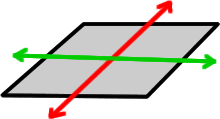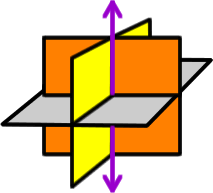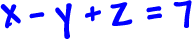So, what the heck is something like?

Well, when we have two variables, x and y, we have a line... and we graph them on a 2-dimensional plane.  So, with three variables, xyand z, we'll be in three dimensions...  That's 3-D space!Pretend that the floor of the room you are in is our old x-y plane...The third dimension will be the vertical line shooting up from the corner of the room.

This is really all you need to worry about... unless you're going to take Calculus 3.  (Which is a very cool class, by the way!  Cough - geek!)

So, an equation likeis a plane that lives in 3-D space.

With a system of three equations and three unknowns, we've got three planes in 3-D space.  Look in the corner of your room again...  That little corner is THE one point where the two walls and the floor (or the ceiling -- depending on where you're looking) intersect.So, hopefully, we'll get a nice (x, y, z) point like (1, -2, 4)for an answer.(Check out the "Freaky Things" lesson!)

This means that x = 1,  y = -2,  z = 4 will work in ALL three of the original equations you started with.